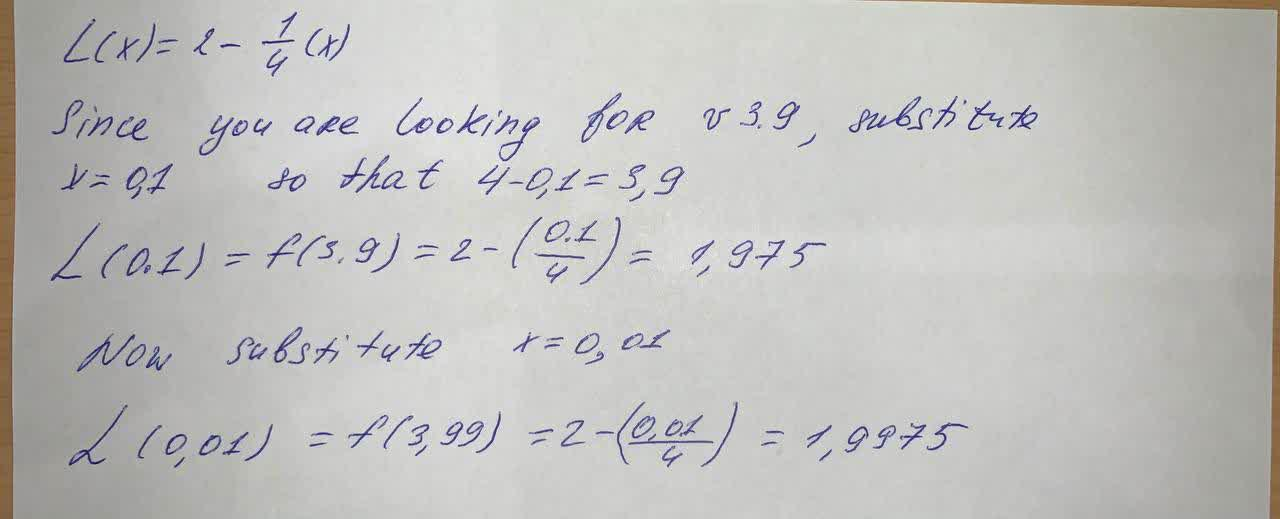Find the linear approximation of the function f(x)=\sqrt{4-x} at a=0Use L(x) to approximate the numbers sqrt (3.9) and sqrt (3.99) Round to four decimal placesabondantQ 2021-06-01 Answered

Find the linear approximation of the function $$f(x)=\sqrt{4-x}$$ at $$a=0$$
Use L(x) to approximate the numbers $$\sqrt{3.9}$$ and $$\sqrt{3.99}$$ Round to four decimal places

• Questions are typically answered in as fast as 30 minutes

Solve your problem for the price of one coffee

• Math expert for every subject
• Pay only if we can solve itestenutCNot exactly what you’re looking for?content_user

$$L(x)=2-\frac{1}{4}(x)$$

Since you are looking for v 3.9, substiute $$x=0,1$$ so that $$4-0,1=3,9$$

$$L(0,1)=f(3.9)=2-(\frac{0.1}{4})=1.975$$

Now substitute $$x=0,01$$

$$L(0,01)=f(3,99)=2-(\frac{0.01}{4})=1.9975$$